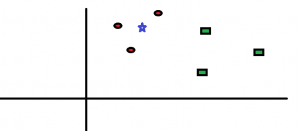# First Machine Learning Project

Scroll this

You might have heard a lot about machine learning and data analysis these days. If you are wondering what are the benefits of Machine Learning and how it is used in real world, then this post is for you. Here I will present to you a very basic and interesting Machine Learning project which you can build yourself. I am sure, this will surely give you a start in Machine Learning with python.

Prerequisites for this project – Installed Python and its modules – Pandas and Scikit-learn.

Lets first discuss the problem statement – Given the mass, height, width and colour of some particular fruit, we want to predict the name of the fruit. For this, we need to already know mass, height, width and colour of different fruits. Using that data we will build our Machine Learning system which can then take up any mass, height, width and colour and will return the name of the fruit.

Here is the sample data –

 fruit_label fruit_name fruit_subtype mass width height color_score 1 apple granny_smith 192 8.4 7.3 0.55 1 apple granny_smith 180 8 6.8 0.59 1 apple granny_smith 176 7.4 7.2 0.6 2 mandarin mandarin 86 6.2 4.7 0.8 2 mandarin mandarin 84 6 4.6 0.79

We have dataset of 60 such rows. You can download it from fruit_data_with_colors. (right click and save this file)

Now, we will be using this dataset to train our system which can predict the name of the fruit from input mass, width and height. This is called Supervised learning.

In Supervised Learning, we split our data set into 2 sets called Training data and Testing data in ratio of 75%/25% i.e. 75% of our data will be used as Training data and rest 25% will be used at Testing data. (out of 60 rows, 45 rows will be used to train our system for prediction and rest 15 rows will be used to test the accuracy of our trained system.)

Step 1: Import modules and read the data

```import numpy as np
import matplotlib.pyplot as plt
import pandas as pd
from sklearn.model_selection import train_test_split

fruit_label fruit_name fruit_subtype mass width height color_score
1 apple granny_smith 192 8.4 7.3 0.55
1 apple granny_smith 180 8.0 6.8 0.59
1 apple granny_smith 176 7.4 7.2 0.60
2 mandarin mandarin 86 6.2 4.7 0.80
2 mandarin mandarin 84 6.0 4.6 0.79

Step 2: Split data into Train and Test data. ‘X’ is input parameters using which prediction is to be made. ‘y’ is the output which is the prediction of fruit name. Following is the way to split the data into default 75%/25% Train-Test.

```X = fruits[['mass', 'width', 'height']]
y = fruits['fruit_label']
X_train, X_test, y_train, y_test = train_test_split(X, y, random_state=0)
```

Step 3: This is the most important step. We will apply K-Nearest Neighbour algorithm of classification. Its pretty easy to understand. Consider the following image.Lets assume red dots represents apples and green dots represents oranges. Lets say, The points are plotted with mass on x-axis and width on y-axis. The red and green points belong to Train dataset. Let say, we got a new point which is marked as star and we don’t know which fruit it is. We only know its mass and width and hence we plotted this point. Now, as per KNN algorithm, the blue star is most similar to red dots (apples) because it is nearest to red dots. KNN uses a variable K which takes up integers. K=1 represents that the one point closest to blue star will be used for its prediction. K=3 will represent that three closest points to blue star will be checked.Hence, the new data point (star) will be predicted as red dot (apple). (You can read more about KNN by clicking here.)

This can be done just with two lines of code using python:

```from sklearn.neighbors import KNeighborsClassifier
knn = KNeighborsClassifier(n_neighbors = 5)
knn.fit(X_train, y_train)```

We can check the accuracy of our using our test data as follows.

`print(knn.score(X_test, y_test))`

It will give 0.53. Which shows that our system is ~53% accurate.

Step 4: Lets now predict the fruit by giving some random mass, width and height as input. First example: a small fruit with mass 20g, width 4.3 cm, height 5.5 cm. This is how we will do prediction using knn.

```fruit_prediction = knn.predict([[20, 4.3, 5.5]])
print(fruit_prediction)```

The output will be a digit ‘2’ which is a fruit_label which corresponds to ‘mandarin’ fruit.

Similarly, now you can predict the fruit by giving any mass, width and height to knn.predict function.

This is the complete code:

```import numpy as np
import matplotlib.pyplot as plt
import pandas as pd
from sklearn.model_selection import train_test_split

lookup_fruit_name = dict(zip(fruits.fruit_label.unique(), fruits.fruit_name.unique()))
print(lookup_fruit_name)

# For this example, we use the mass, width, and height features of each fruit instance
X = fruits[['mass', 'width', 'height']]
y = fruits['fruit_label']
# default is 75% / 25% train-test split
X_train, X_test, y_train, y_test = train_test_split(X, y, random_state=0)

from sklearn.neighbors import KNeighborsClassifier
knn = KNeighborsClassifier(n_neighbors = 5)
knn.fit(X_train, y_train)
print(knn.score(X_test, y_test))

# first example: a small fruit with mass 20g, width 4.3 cm, height 5.5 cm
fruit_prediction = knn.predict([[20, 4.3, 5.5]])
print(lookup_fruit_name[fruit_prediction])

# second example: a larger, elongated fruit with mass 100g, width 6.3 cm, height 8.5 cm
fruit_prediction = knn.predict([[100, 6.3, 8.5]])
print(lookup_fruit_name[fruit_prediction])

plot_fruit_knn(X_train, y_train, 5, 'uniform') # we choose 5 nearest neighbors
```

I hope with this post you have got a basic idea about what exactly Machine Learning is and how it is used in real world.

Signup now and receive an email once we publish new content.

I agree to have my personal information transfered to MailChimp ( more information )